# Surface Area of 3D Shapes

GCSELevel 4-5AQACambridge iGCSEEdexcelOCRWJEC

## Surface Area

The surface area of a 3D shape is a measure of how much area the surfaces of that shape have in total.

Shapes that you have to work out the surface area of can fall into one of two categories.

– All the faces are flat – e.g. square or rectangle.

– Some/all of the faces are curved –  e.g. cone or sphere.

Make sure you are happy with the following before continuing:

Level 4-5GCSEAQAEdexcelOCRWJECCambridge iGCSE

## Surface Area of Cubes and Cuboids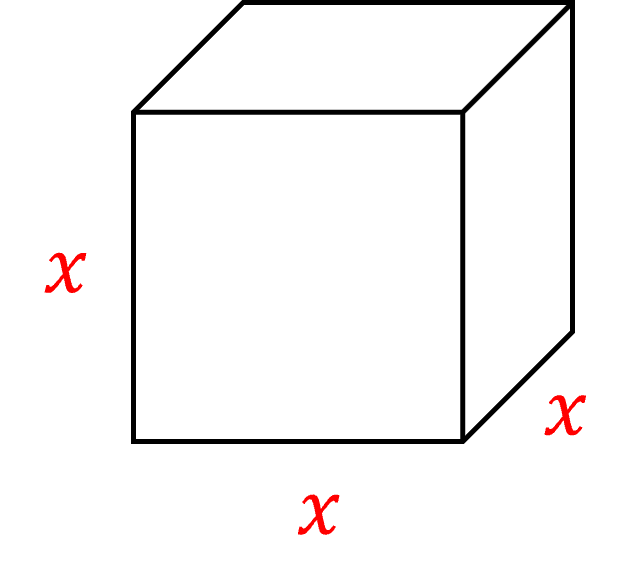A cube is made of $\textcolor{blue}{6}$ identical square faces.

If a cube has a side length of $\textcolor{red}{x}$, then each of these faces are squares with side lengths $\textcolor{red}{x}$.

So the area of one face is $\textcolor{red}{x}\times\textcolor{red}{x}=\textcolor{red}{x}^{2}$

Hence, the surface area of the whole cube is $\textcolor{blue}{6}$ times this, or $\textcolor{blue}{6}\textcolor{red}{x}^{2}$

$\text{Surface Area of a Cube}=\textcolor{blue}{6}\textcolor{red}{x}^{2}$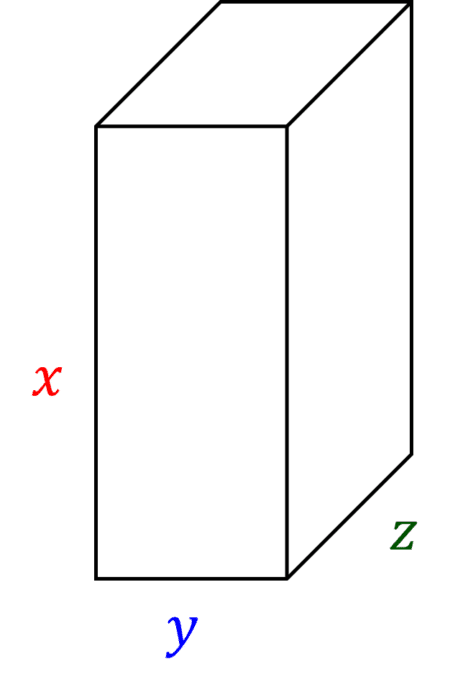A cuboid is made of $6$ rectangular faces.

Opposite rectangular faces are equal, meaning that we have $3$ pairs of differently sized rectangles.

If the cuboid has sides $\textcolor{red}{x}$, $\textcolor{blue}{y}$ and $\textcolor{green}{z}$, then…

Two of the rectangles have sides $\textcolor{red}{x}$ and $\textcolor{blue}{y}\\$

Two of the rectangles have sides $\textcolor{red}{x}$ and $\textcolor{green}{z}\\$

Two of the rectangles have sides $\textcolor{blue}{y}$ and $\textcolor{green}{z}$

This gives rectangle areas of $\textcolor{red}{x}\textcolor{blue}{y}$, $\textcolor{red}{x}\textcolor{green}{z}$ and $\textcolor{blue}{y}\textcolor{green}{z}$

Since there are $2$ of each of these rectangles, the total surface area is $2(\textcolor{red}{x}\textcolor{blue}{y}+\textcolor{red}{x}\textcolor{green}{z}+\textcolor{blue}{y}\textcolor{green}{z})$

$\text{Surface Area of a Cuboid}=2(\textcolor{red}{x}\textcolor{blue}{y}+\textcolor{red}{x}\textcolor{green}{z}+\textcolor{blue}{y}\textcolor{green}{z})$

Level 4-5GCSEAQAEdexcelOCRWJECCambridge iGCSE

## Surface Area of a Cone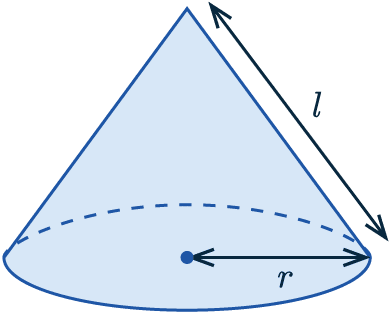Surface area $= \pi \textcolor{blue}{r}\textcolor{red}{l} + \pi \textcolor{blue}{r}^2$

Where $\textcolor{blue}{r}$ is the radius of the cone.

$\textcolor{red}{l}$ is the slanted height of the cone.

Level 4-5GCSEAQAEdexcelOCRWJECCambridge iGCSE

## Surface Area of a Cylinder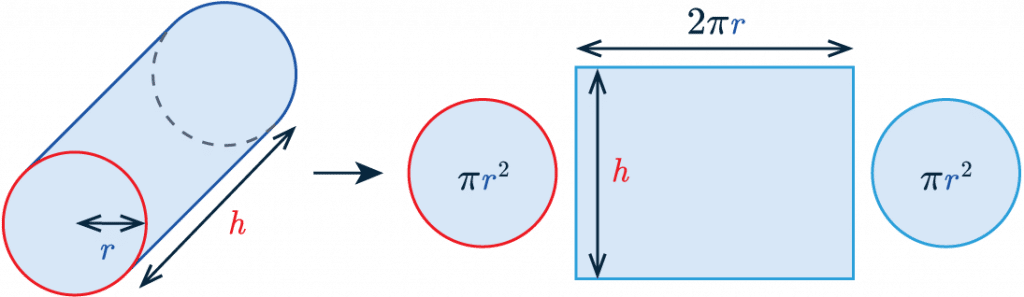Surface area $= 2\pi \textcolor{blue}{r} \textcolor{red}{h} + 2 \pi \textcolor{blue}{r}^2$

Where $\textcolor{blue}{r}$ is the radius of the cylinder.

$\textcolor{red}{h}$ is the height of the cylinder.

Level 4-5GCSEAQAEdexcelOCRWJECCambridge iGCSE

## Surface Area of a Sphere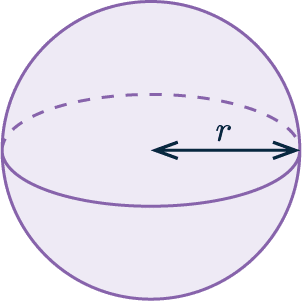Surface area $= 4\pi \textcolor{blue}{r}^2$

Where $\textcolor{blue}{r}$ is the radius of the sphere.

Level 4-5GCSEAQAEdexcelOCRWJECCambridge iGCSE
Level 4-5GCSEAQAEdexcelOCRWJECCambridge iGCSE

## Example 1: Surface Area of a Square Based Pyramid

Find the surface area of the square based pyramid shown:

[3 marks]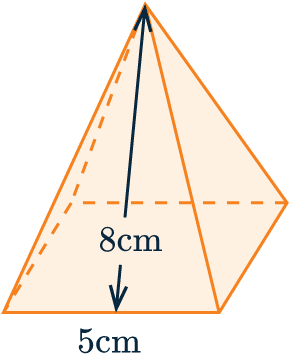All $4$ of its triangular faces are identical.

We only need to work out the area of one and then multiply it by $4$ to get the area of them all.

Area of a triangle is $\frac{1}{2}bh$, and here the base is $5$ cm and the height is $8$cm.

$\dfrac{1}{2}\times5\times8=20\text{ cm}^2$

Therefore, the area of all $4$ triangles is

$4\times20=80\text{ cm}^2$.

Next we need to add the area of the square base to the total area.

Area of base = $5^2=25\text{ cm}^2$

Therefore,

Total surface area $=25+80=105\text{ cm}^2$

Level 4-5GCSEAQAEdexcelOCRWJECCambridge iGCSE

## Example 2: Surface Area of a Cylinder

Find the surface area of the cylinder, giving your answer to $4$ significant figures.

[3 marks]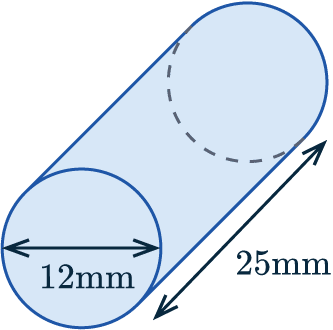We know the formula for the surface area of a cylinder is

Surface area = $2\pi r h + 2\pi r^2$

From the diagram we can see:

– the radius, r, = $12\div 2 = 6$ mm

– the height of the cylinder is $25$ mm

We can then apply these to the equation:

$(2 \times \pi \times 6 \times 25 ) + ( 2 \times \pi \times 6^2)$

Surface area = $300\pi + 72\pi = 1169 \text{ mm}^2$

Level 4-5GCSEAQAEdexcelOCRWJECCambridge iGCSE

## Example 3 : Surface Area of a Cone

Calculate the surface area of the cone shown:

[3 marks]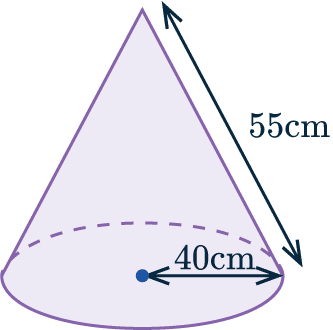The formula for the surface area of a cone is

Surface area of a cone = $\pi r^2+\pi rl$

Where $r$ is the radius of the base and $l$ is the slant height.

Therefore, the surface area of this cone is

$\pi\times 40^2+\pi\times40\times55=11938.1\text{ cm}^2\text{ (1 dp)}$

Level 4-5GCSEAQAEdexcelOCRWJECCambridge iGCSE

## Example 4: Surface Area of a Sphere

A sphere with surface area $1,025\text{ m}^2$ is shown.

Work out the length of the radius.

Give your answer to $2$ significant figures.

[3 marks]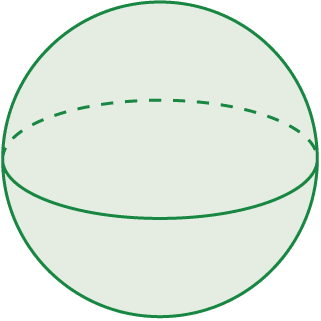The formula for the surface area of a sphere is

Surface area of a sphere $\, = 4\pi r^2$

We know that the surface area is $1025$ m$^2$, so we can set the formula above equal to $1025$ to get

$4\pi r^2=1025$

Then, if we divide both sides by $4\pi$, we get

$r^2=1025\div4\pi=81.5669...$

Finally, square rooting this we get

$r=\sqrt{81.5669...}=9.0\text{ m} \text{ (2 sf)}$

Level 4-5GCSEAQAEdexcelOCRWJECCambridge iGCSE

## Surface Area of 3D Shapes Example Questions

A cuboid has 6 flat, rectangular faces, and we will need the areas of all of them. Specifically, there are 3 pairs of faces since the front and back faces are the same, the top and bottom faces are the same, and the left and right faces are the same.

Firstly, the front face is a rectangle with height 4mm and width 2.5mm, so it has area

$4\times2.5=10\text{ mm}^2$

Therefore, the back face also has area $10\text{ mm}^2$.

The face on the right has length 6mm and height 4mm, so it has area

$6\times4=24\text{ mm}^2$

Therefore, the left face also has area $24\text{ mm}^2$

The face on the top has length 6mm and width 2.5mm, so it has area

$6\times2.5=15\text{ mm}^2$

Therefore, the bottom face also has area $15\text{ mm}^2$.

Thus, adding all these values together, we get the total surface area to be

$10+10+24+24+15+15=98\text{ mm}^2$Gold Standard Education

We know the whole surface area is $120\text{cm}^2$ and we also know the radius. To work out the slant height, we need to first work out what the curved surface area is. In other words, we need to subtract the surface area of the base of the cone (since that’s the only other face) from 120 to get the curved surface area. The base is a circle, so its area is:

$\pi \times 3^2=9\pi\text{ cm}^2$

Subtracting this from the total we have

$120-9\pi=91.725...$

This is written as a decimal to give an idea of how big it is, but when you put it into your calculator you should either use the ANS key to store the value, or you should directly type in $120-9\pi$. If you don’t, it could affect your final answer.

Now, this must the area of the curved face, and the formula for the area of the curved face is given to us: $\pi rl = 3\pi l$ (since we know $r=3$). So, setting this formula equal to the value we worked out, we get

$3\pi l=120-9\pi$

Then, to find the slant height, we will divide both sides by $3\pi$ to get

$\dfrac{120-9\pi}{3\pi}=9.7\text{ cm (1dp)}$.Gold Standard Education

We need to firstly work out the surface area of the sphere. So, with $r=8.5$, we get

$\text{surface area }=4\times\pi\times(8.5)^2=907.920...\text{ m}^2$

Now, since each pot covers ten square metres, we must divide this value by 10 to see how many pots he will require:

$907.920... \div 10=90.720...$

Since he can’t buy exactly 90.720… pots, he will have to buy 91 to cover the whole sphere. Therefore, the cost of all his paint will be

$91\times 9.60=\pounds873.60$

That’s a lot of paint.Gold Standard Education

The area of the two triangular faces is,

$\text{area}=2\times \dfrac{1}{2}\times 6\times 8 = 48\text{ cm}^2$

The area of the rectangular base is,

$\text{area}=6\times 11 = 66 \text{ cm}^2$

To find the area of the two sides we have to first find the slanted height of the prism:

$\text{AB}=\sqrt{8^2+3^2} =\sqrt{73}$

Hence, the area of the two sides are:

$\text{area}=2\times 11\times \sqrt{73}$

Total surface area:

$\text{surface area }=48+66+\sqrt{73}= 301.97\text{ cm}^2$Gold Standard Education

The area of the square base is,

$\text{area}=12\times12=144\text{cm}^2$

Finding the length of the midpoint of DC to E:

$\sqrt{10^2+6^2} = \sqrt{136}$

Hence the area of the 4 triangular faces is,

$4\times\dfrac{1}{2}\times12\times\sqrt{136}= 279.89\text{cm}^2$

Total surface area:

$\text{surface area}=279.89+144= 423.89\text{ cm}^2$Gold Standard Education

## Surface Area of 3D Shapes Worksheet and Example Questions

### (NEW) Surface Area Exam Style Questions - MME

Level 4-5Level 6-7GCSENewOfficial MME

## Surface Area of 3D Shapes Drill Questions

### Surface Area

Level 4-5GCSE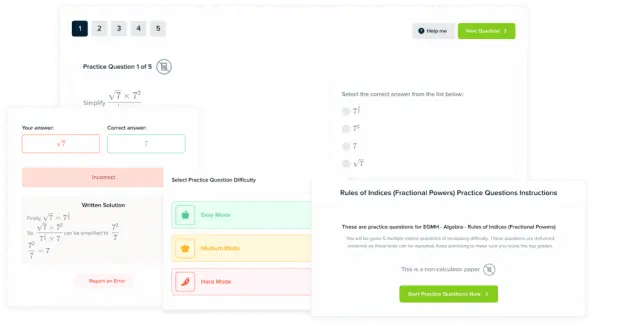Product

### £19.99

/month

Learn an entire GCSE course for maths, English and science on the most comprehensive online learning platform. With revision explainer videos & notes, practice questions, topic tests and full mock exams for each topic on every course, it’s easy to Learn and Revise with the MME Learning Portal.

Level 1-3KS3

Level 4-5KS3